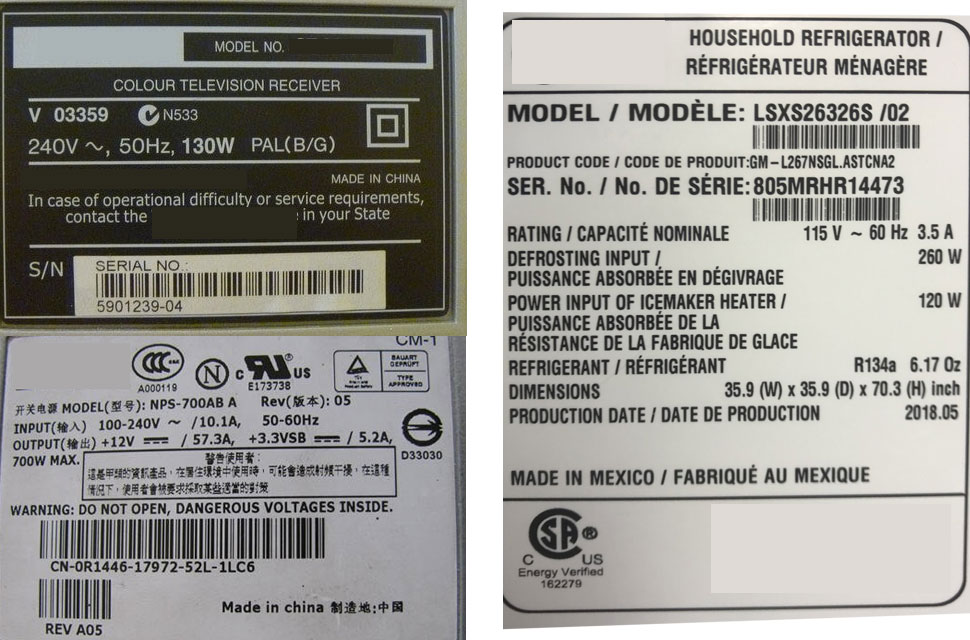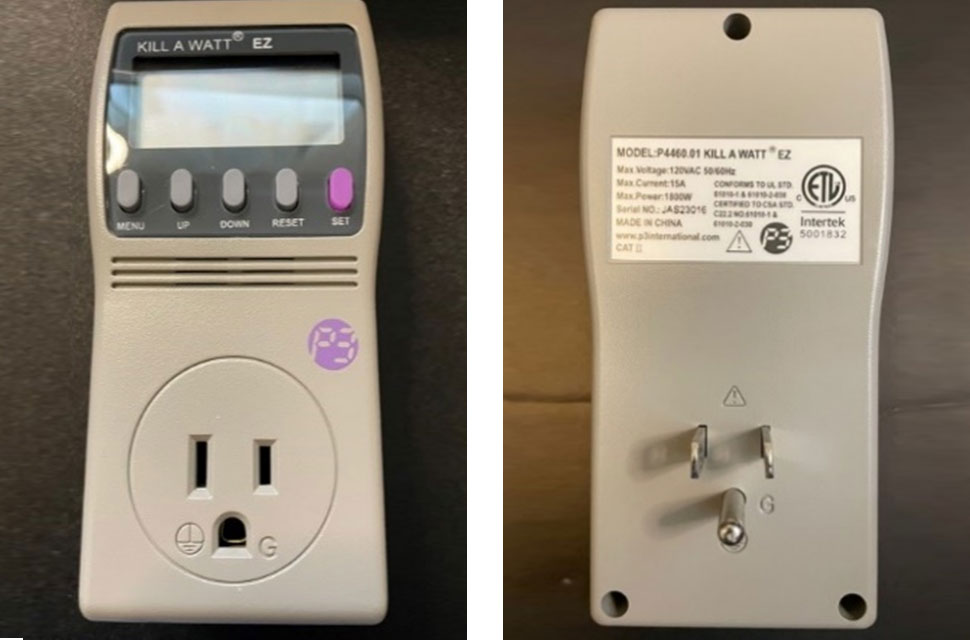Have you found the books Clark gave to elementary school libraries? This year’s book is Drop: An Adventure through the Water CycleActivity

# Calculate Consumption

< Back to All Games & Activities

## Instructions

Ready to get started with this activity? To keep track of your progress, check off the instructions for each step below as they are completed. Make sure to check the box of the last step when you’re done to receive congratulations for your completed activity!

### Consider this:

Have you ever wondered how much it costs to have a refrigerator, or a gaming console plugged in? In this activity you will start by manually calculating the cost of an appliance or appliances, and after you will check the results using a watt meter from Clark Public Utilities.

Above, you’ll see a button titled “Calculation Table.” You’ll need to download that spreadsheet to use for this activity.

An appliance or device’s rating can typically be found on a label on the back or underneath the device. Keep in mind that sometimes only the amperage will be listed. When that’s the case, multiply the number of amps by 120 volts to get the watts (Amps x 120 Volts = Watts).• Enter the wattage of the appliance or device into the Calculation Table spreadsheet that you downloaded.
• Next, enter the number of hours per day that you use the device. (Some appliances, like refrigerators, run continuously. However, they do not use the rated wattage at all times. A Watt Meter is a great device to use to calculate the actual energy usage that these appliances use.)
• To find the annual cost, enter the number of days per year that you typically use the appliance or device. (Enter 1 if you want to calculate the daily cost, 30 for the monthly cost or 365 if you use the device daily.)
• The cost of 1 kWh in Clark County is \$0.0816. 1 kilowatt hour (kWh) is a 1000 watt device running for 1 hour, or a 100 watt device running for 10 hours. Based on the information you input below, you can manually calculate the estimated cost for the device.
• Plug the Watt Meter into the wall, and the appliance/device into the Watt Meter. The Watt Meter will now start recording the amount of time the device was plugged in and how much electricity it uses.
• Leave the device plugged in for a few days to get a more accurate result of actual usage.
• Before you unplug the Watt Meter from the wall, record the number of hours that the device was plugged in and the amount of electricity that was consumed. This is measured in kilowatt hours (kWh). To locate this data, press the pink button on the Watt Meter and record them on the Watt Meter line in the spreadsheet.• A watt is the basic unit of measurement used for electrical, thermal, and mechanical power.
• Clark Public Utilities calculates the electric bill based on how many kilowatt-hours or units of energy are used each month.
• Can you estimate the total household electric bill based on items using power in your home? This would be tough! We bet you can calculate a pretty close estimate to show much electricity is used in your bedroom by knowing the watts and hours of use.

Clark Public Utilities website has some calculators to help with estimations:

?Questions?